#### Tarski sentences

Recall the logical predicates that were formed in Section 3.1. They will be used again here, but now they are defined with a little more flexibility. For any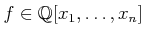, an atom is an expression of the form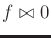, in which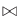may be any relation in the set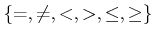. In Section 3.1, such expressions were used to define logical predicates. Here, we assume that relations other than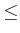can be used and that the vector of polynomial variables lies in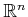.

A quantifier-free formula,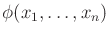, is a logical predicate composed of atoms and logical connectives, and,'' or,'' and not,'' which are denoted by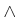,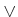, and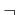, respectively. Each atom itself is considered as a logical predicate that yields TRUE if and only if the relation is satisfied when the polynomial is evaluated at the point.

Example 6..2 (An Example Predicate)   Let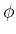be a predicate over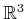, defined as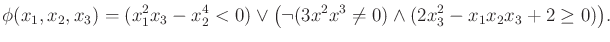(6.8)

The precedence order of the connectives follows the laws of Boolean algebra.Let a quantifier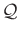be either of the symbols,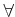, which means for all,'' or, which means there exists.'' A Tarski sentence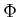is a logical predicate that may additionally involve quantifiers on some or all of the variables. In general, a Tarski sentence takes the form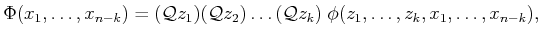(6.9)

in which the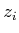are the quantified variables, the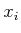are the free variables, andis a quantifier-free formula. The quantifiers do not necessarily have to appear at the left to be a valid Tarski sentence; however, any expression can be manipulated into an equivalent expression that has all quantifiers in front, as shown in (6.9). The procedure for moving quantifiers to the front is as follows : 1) Eliminate any redundant quantifiers; 2) rename some of the variables to ensure that the same variable does not appear both free and bound; 3) move negation symbols as far inward as possible; and 4) push the quantifiers to the left.

Example 6..3 (Several Tarski Sentences)   Tarski sentences that have no free variables are either TRUE or FALSE in general because there are no arguments on which the results depend. The sentence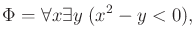(6.10)

is TRUE because for any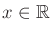, some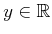can always be chosen so that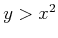. In the general notation of (6.9), this example becomes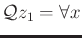,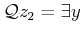, and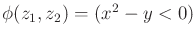.

Swapping the order of the quantifiers yields the Tarski sentence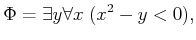(6.11)

which is FALSE because for any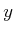, there is always ansuch that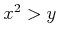.

Now consider a Tarski sentence that has a free variable: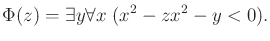(6.12)

This yields a function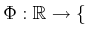TRUE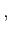FALSE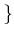, in which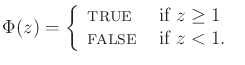(6.13)

An equivalent quantifier-free formulacan be defined as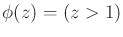, which takes on the same truth values as the Tarski sentence in (6.12). This might make you wonder whether it is always possible to make a simplification that eliminates the quantifiers. This is called the quantifier-elimination problem, which will be explained shortly.Steven M LaValle 2020-08-14